# Implementing the Pipe Operator in TypeScript

## What is the pipe operator?

The pipe operator is used to chain function calls together in a more readable and concise way. The operator takes the output of one function as the input for the next function in the chain. Here’s an example of pipes in Elixir.

``````"hello"
|> String.upcase() # turn string into uppercase
|> String.reverse() # reverse the string returned from `String.upcase()`
|> IO.puts() # print the output from `String.reverse()`

# "OLLEH"``````

In the example above, the pipe operator `|>` takes the output of the previous function and passes it as the input for the next function. Here’s a more complex example. It’s my solution to Advent of Code 2022 day 1 pt.2.

``````File.read!("./input.txt")
|> String.split("\n\n")
|> Enum.map(fn elf ->
elf
|> String.split("\n")
|> Enum.map(fn e -> e |> String.to_integer() end)
|> Enum.sum()
end)
|> Enum.sort(:desc)
|> Enum.slice(0..2)
|> Enum.sum()
|> IO.inspect()``````

You can see how the pipe operator really helps in improving the readability of the code. The code reads like a sentence. Pipes also eliminate the need to create temporary variables to store the output of each function call or using nested function calls. As a result developers also don’t have to worry about naming variables which is my least liked part of programming.

## Implementing the pipe operator in TypeScript

After watching Theo’s video on the proposal for adding pipes in JavaScript, I was inspired to implement the pipe operator as a function that composes functions together. Here is what I set out to achieve.

``````const len = (s: string): number => s.length;
const double = (n: number): number => n * 2;
const square = (n: number): number => n ** 2;

console.log(pipe("hi", len, double, square)); // 16``````

### Initial implementation

This was my first iteration of the `pipe` function.

``````const pipe = (value: any, ...fns: Function[]) =>
fns.reduce((acc, fn) => fn(acc), value);``````

Here’s what this code is doing:

• The function takes two arguments: `value` of any type, and `fns` which is an array of functions. The spread operator `...` allows for any number of functions to be passed in.
• The `reduce` function is used to iterate over the array of functions and pass the output of the previous function as the input for the next function.
• The callback function passed to `reduce` takes two arguments: `acc` which is the accumulator, and `fn` which is the current function in the array. The first function in the array is applied to the initial value, which is the `value` argument passed to the function. Each subsequent function is applied to the output of the previous function, chaining them together.
• It then returns the output of the last function in the array.

And this works!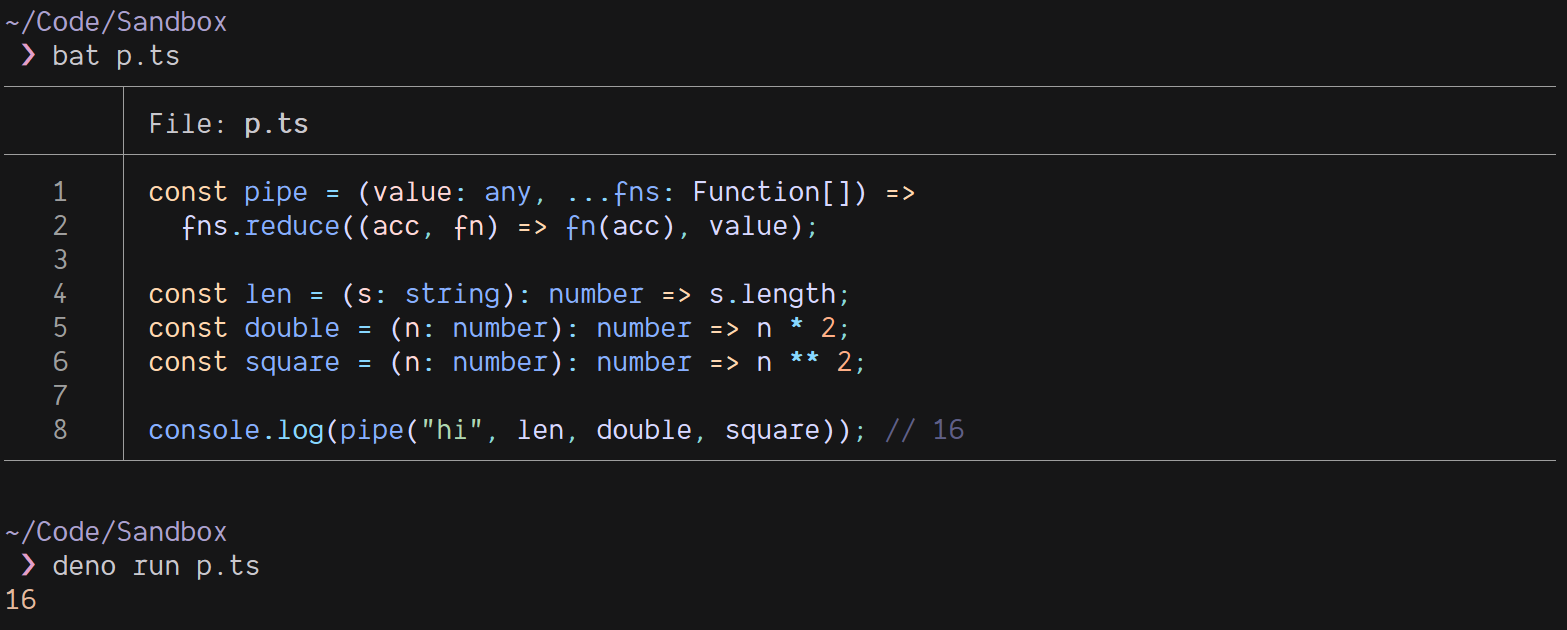### Problems with the initial implementation

There are a few problems with this implementation. The first problem that I immediately noticed is that the type of the returned value from the function is `any`.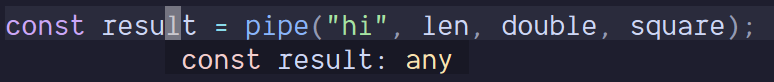Obviously this is not good. Ideally this type should be inferred by the TypeScript compiler. I got some help from Reddit and a user there suggested this:

``````type Fn = (...args: any[]) => any;

type LastReturnType<L extends Fn[]> = L extends [...any, infer Last extends Fn]
? ReturnType<Last>
: never;

const pipe = <Funcs extends Fn[]>(value: any, ...fns: Funcs) =>
fns.reduce((acc, fn) => fn(acc), value) as LastReturnType<Funcs>;``````

This is definitely some crazy TypeScript. Let’s break it down.

• `Fn` is a type alias for a function that takes any number of arguments of `any` type and returns `any` type.
• `LastReturnType` is a generic type that takes an array of functions and returns the return type of the last function in the array. The `infer` keyword is used to infer the type of the last function in the array.
• It uses variadic tuple types to know what the last function in the tuple is and then uses the `ReturnType` type utility to get the return type of the last function.
• Then the `pipe` function is defined as a generic function that takes an initial value of any type and an arbitrary number of functions of the `Fn` type. It then casts the return value of the function to the return type of the last function in the array.

So now the type of the returned value is inferred correctly.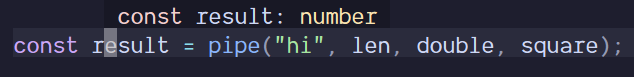But there’s still a problem. Let me demonstrate it with an example.

``````// does not show an error
const result = pipe("hi", double, len, square);

// Argument of type 'number' is not assignable to parameter of type 'string'.
const result2 = square(len(double("hi")));``````

Here we’re trying to double the string “hi”, which is impossible as we’re passing a string to a function that expects a number. You can see we get the appropriate error when we try to do this by nesting the function calls. But our `pipe` function does not show any errors.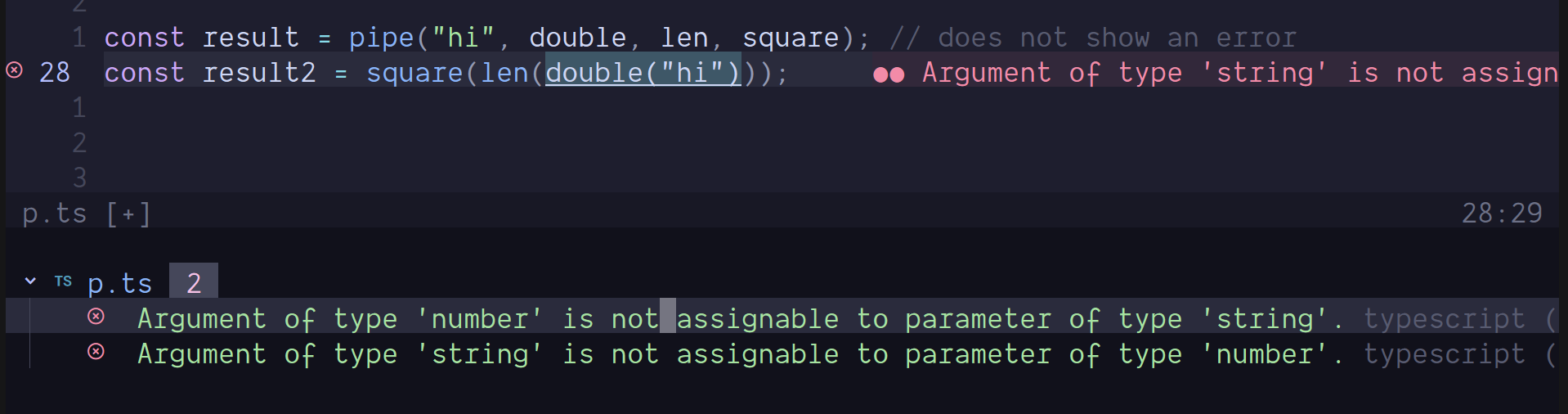This is a perfect use case for Higher Kinded Types. Unfortunately, TypeScript does not support Higher Kinded Types yet. There isn’t a way to say “for all these functions, the input type is contravariant to the output type of the previous function”.

### So what’s the solution?

Turns out, the easiest and most straightforward solution is to set an upper bound on the number of functions that can be passed to the `pipe` function and use function overloading to define the type of the returned value.

``````function pipe<A>(value: A): A;
function pipe<A, B>(value: A, fn1: (input: A) => B): B;
function pipe<A, B, C>(value: A, fn1: (input: A) => B, fn2: (input: B) => C): C;
function pipe<A, B, C, D>(
value: A,
fn1: (input: A) => B,
fn2: (input: B) => C,
fn3: (input: C) => D
): D;
function pipe<A, B, C, D, E>(
value: A,
fn1: (input: A) => B,
fn2: (input: B) => C,
fn3: (input: C) => D,
fn4: (input: D) => E
): E;
// ... and so on

function pipe(value: any, ...fns: Function[]): unknown {
return fns.reduce((acc, fn) => fn(acc), value);
}``````

This might seem very manual but it’s definitely the best way to do this. It works very well and also gives fairly easy to understand type errors for the user. Let’s see how it works.

The first five function declarations are overloads of the `pipe` function, each one of them has a different set of parameters, each overload corresponds to a different number of functions that can be passed to the `pipe` function.

• The first overload takes a single argument of generic type `A` and returns the same value without applying any function to it.
• The second overload takes two arguments, value of type `A`, and `fn1` a function that takes an argument of type `A` and returns a value of type `B`.
• The third overload takes three arguments, value of type `A`, `fn1` a function that takes an argument of type `A` and returns a value of type `B`, and `fn2` a function that takes an argument of type `B` and returns a value of type `C`.
• There can be any number of overloads, but I’ve only defined five for demonstration purposes.

Each overload corresponds to a different number of functions, and each function’s input type is the output of the previous function, this way the `pipe` function will not only return the output type of the last function passed but also type check the input and output types of each function in the pipeline.

The last function declaration is the actual implementation of the `pipe` function. It takes an initial value of any type and an arbitrary number of functions and applies them to the initial value in the order they are passed. The logic is the same as before.

The advantage of this implementation is that it allows the `pipe` function to be more type-safe as it ensures that the input and output types of each function in the pipeline are consistent and match the type of the initial value, and it also ensures that the functions passed to the `pipe` function have the correct signature.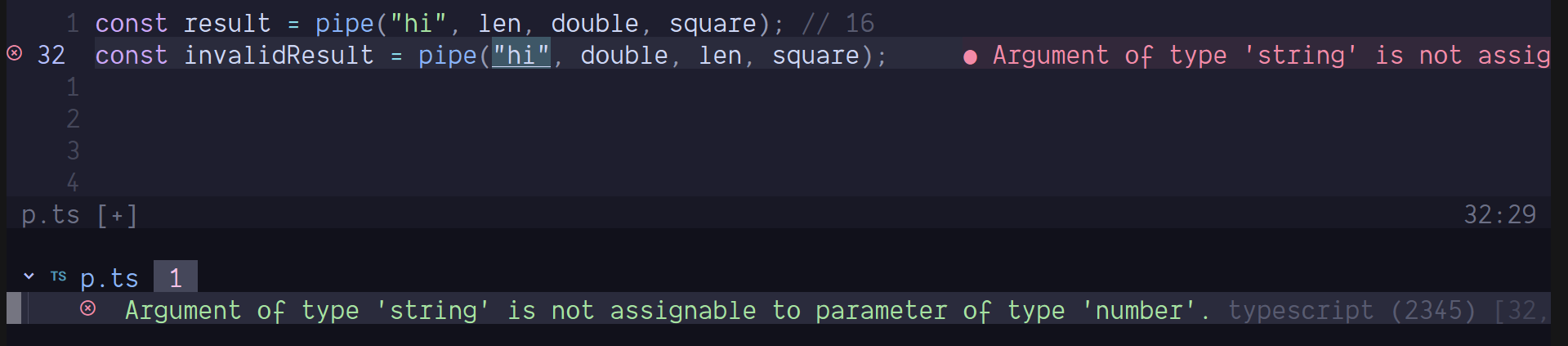I love my arrow functions and almost never use the `fuction` keyword. Unfortunately, we can’t use arrow functions for overloading. We can only use the `function` keyword for overloading. But what we can do is implement the overloads in an interface and then implement the actual function as a function of that interface.

``````interface Pipe {
<A>(value: A): A;
<A, B>(value: A, fn1: (input: A) => B): B;
<A, B, C>(value: A, fn1: (input: A) => B, fn2: (input: B) => C): C;
<A, B, C, D>(
value: A,
fn1: (input: A) => B,
fn2: (input: B) => C,
fn3: (input: C) => D
): D;
<A, B, C, D, E>(
value: A,
fn1: (input: A) => B,
fn2: (input: B) => C,
fn3: (input: C) => D,
fn4: (input: D) => E
): E;
// ... and so on
}

const pipe: Pipe = (value: any, ...fns: Function[]): unknown => {
return fns.reduce((acc, fn) => fn(acc), value);
};``````

This is same as the previous implementation, but we’re using an interface to implement the overloads instead of using the `function` keyword. This way we can use arrow functions for the actual implementation. Pretty neat, right? Everything still works!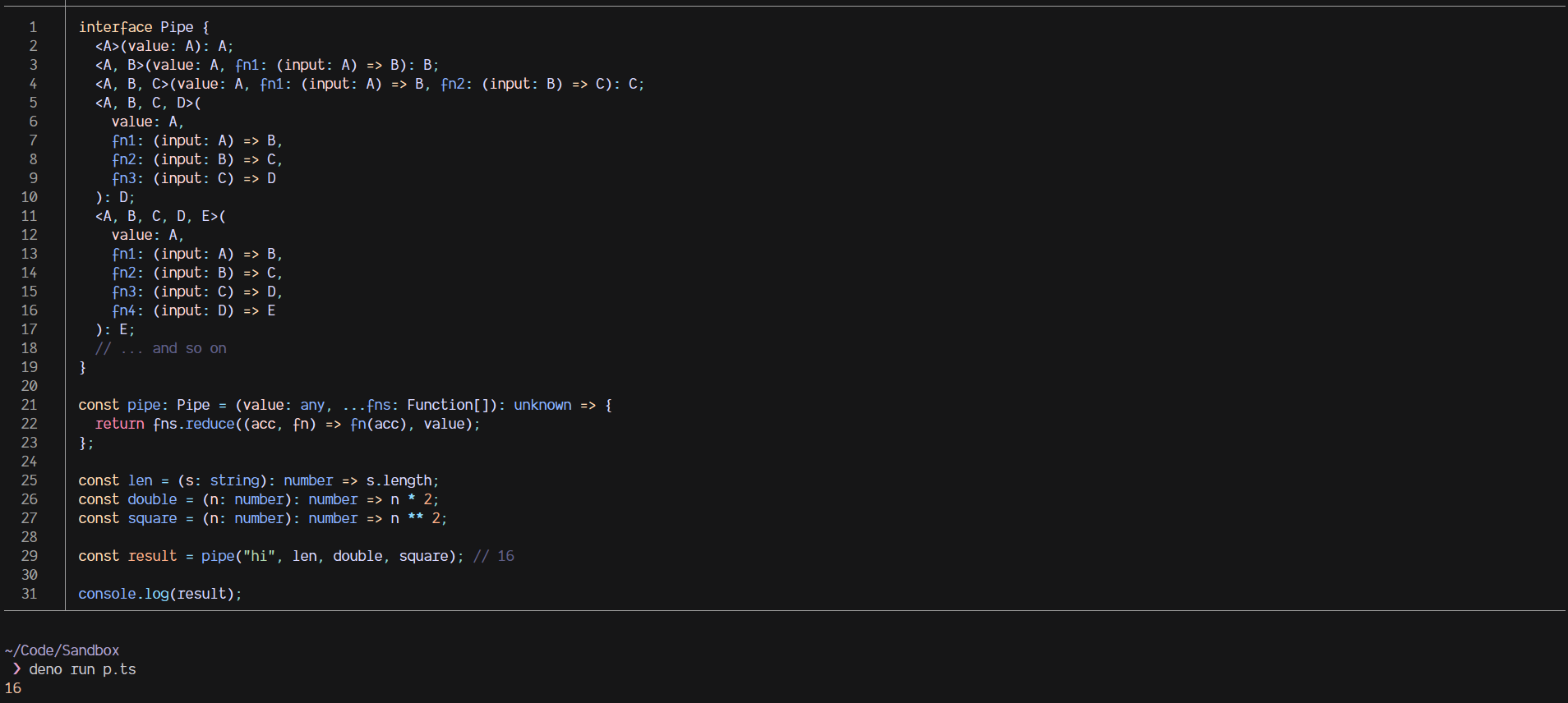## Conclusion

I hope you enjoyed this article. I’ve learned a lot while writing it, and I hope you did too. Thanks for reading!